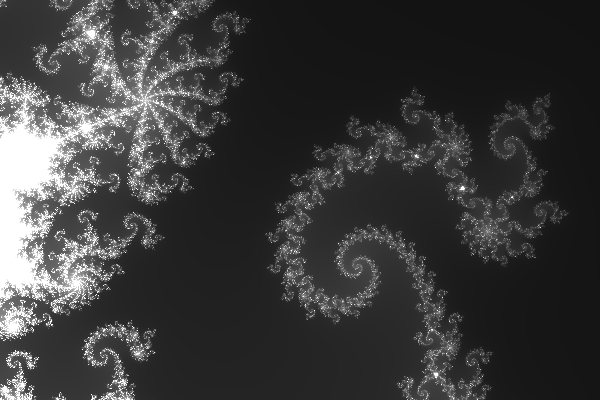# The Mandelbrot curve

The Mandelbrot curve became very trendy in the mid-1980's, soon after its discovery by Benoit Mandelbrot. It was popular both because it looked nice and because it was moderately challenging for programmers to create on the low-power home computers available at that time. Since then it has become less well-known but today's PC's can display it very quickly.

It is generated by a very simple formula applied repeatedly. Start with a complex number z = 0 and a complex offset c = a + bi. On each step of a loop, square z and add c.

z -> z2 + c

Repeat the loop until either the square of z exceeds a moderately large cut-off value, say 100, or a set maximum number of iterations is reached, say 256. Plot at Cartesian position (a, b) the number of iterations of the loop when either of the end conditions is reached. If we interpret the plotted number as brightness in an image then the Mandelbrot curve is seen as the extremely convoluted boundary between regions in which the two types of end condition apply. For values of c inside the Mandelbrot curve, z remains trapped. For values outside the curve, z eventually heads off towards infinite length and never gets smaller.

If the complex numbers z and c are represented as vectors on a 2-axis graph, plotting imaginary part against real part, the repeated operation can be thought of as doubling the angle of the vector z as measured from the x-axis and then adding a constant offset vector c. Cut off occurs when the length of z becomes very large. The behaviour of z under this operation is chaotic along the Mandelbrot curve. It rapidly becomes unpredictable and shows mathematical properties typical of systems in chaos. Such properties were also discovered in the last decades of the 20th century. Their discovery was facilitated by the rise of computing power of course. The characteristic shape of the curve would be a revealing sign to an alien that we have reached a certain level of technology.

Do you want to know what it looks like? Download and run GRIP, then select "New Mandelbrot curve" from the file menu. When you get the image of the whole shape, click on it with the mouse to zoom in to any point. Doing that repeatedly reveals the intricate details of the curve. There is eventually a limit to how far you can zoom in because of the accuracy of the computer calculations.

In Java terms, the image is generated somewhat like this (with suitable scale factors to fill the screen):

double zx = 0, zy = 0;

for (int y = 0; y < imageHeight; y++)
{
double cy = y * scale;

for (int x = 0; x < imageWidth; x++)
{
double cx = x * scale;

for (int n = 0; n < maxLevel; n++)
{
zx2 = zx * zx;
zy2 = zy * zy;

if (zx2 + zy2 > cutOff) break;

zy = 2 * (zx2 - zy2) + cy;
zx = zx2 + zy2 + cx;
}

image.setPixel (x, y, n);
}
}

OK, this is what part of the Mandelbrot curve looks like, as drawn by GRIP:The Mandelbrot curve is also interesting from a philosophical point of view. It does not exist in the physical world nor is it an invention of the human mind but it very definitely exists in a mathematical sense. It has always existed in mathematical space, waiting for us to discover it. It indicates that there really is a mathematical space independent both of human consciousness and of the physical universe.

It is also worth noting that digital computer depictions of the curve can only ever be approximations, limited by the number of bits used for the numbers in the calculations. The exact version only exists in mathematical space. This aspect is related to Gregory Chaitin's ideas about the relationship between mathematics and computing; see for example Chaitin's 2005 book "Meta Maths - The Quest for Omega" available as a paperback from Atlantic Books, London.

Next page